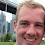## Monday, March 23, 2009

### Adjacency matrix of a tree..

Is there a purely algebraic characterization of the adjacency matrix of a tree ? In other words, given an n X n boolean matrix, can I determine whether it's the adjacency matrix of a tree with purely algebraic conditions (rather than writing down the induced graph and checking).

The reason I'd like this is because I want to talk about the space of such matrices, and I'd like to speak algebraically, rather than indirectly via a conversion to a graph. This seems like something that should be fairly well known: I've found some descriptions that involve all determinants of minors, and was hoping there was something more "compact".

1.There is an algebraic description in terms of the Laplacian matrix, which is almost the same as the adjacency matrix except that the diagonal contains the negative vertex degree instead of 0 (see Wikipedia). The multiplicity of the eigenvalue 0 counts the connected components, and the trace of this matrix is -2 times the number of edges of the graph. So for a tree there must be n-1 nonzero eigenvalues and the trace must be 2n-2 where n is the number of vertices.

2.You might look at the linear algebra literature for what types of matrices can be quickly inverted. I believe trees matrices have been identified as such a class and the related literature may hold a useful characterization. Sorry I can't give a more specific reference.

Jeff P

3.How about the sum of 1's being 2(n-1) and the n^th power having strictly positive entries?

4.can't you just invoke the matrix-tree theorem to count the number of spanning trees and check if it's equal to one?

5.tree = Exactly 1 path between each pair of nodes.

A^k_{i,j} = # of paths between i and j of length k.

I + A + A^2 + .. + A^{n-1} = J (the matrix of all 1's
= (1 - A^n)/(1 - A)

Is that what you're looking for?

6.Suresh, trivial necessary conditions:

1. the sum of all elements must be 2(n-1) (twice the number of eddges)?
2. each row/column must sum >0

I need to refine this, but to borrow some theory from from graph reachability, I think we should have that 0 < b_{ij} <= n for all b_{ij}, where B=(A+A^2...+A^n)

For the loop case, the sum for some pair of nodes will be >n. I not sure this handles disjoint subgraphs.

7.Mitch, trees will have multiple paths between pairs of nodes. They will have only one *simple* path, but your formula counts paths (i.e., it is wrong).

8.There are several things that can be checked algebraically (reusing ideas from several other posts):

i) the number of edges, via the sum of entries of A.

For a tree it must be n-1.

ii) Connectedness.
A^k has a positive (i,j) entry iff there is a path from i to j with k edges, possibly going back and forth repeatedly and using the same edge several times.

A^n is not positive for a tree, as a tree is bipartite, and in exactly n steps you cannot reach every vertex j from every vertex i, only those in the same or the other bipartition class, according to the parity of n.

But for a tree, we have A^n+ A^(n-1) positive, or (I+A)^n positive.

iii) the number of spanning trees, as the determinant of the Laplacian matrix from which one row and column has been removed. (with appropriate sign if not the SAME row and column are removed), by the matrix-tree theorem.
For a tree this number is 1.

Condition (iii) is necessary and sufficient: a graph with exactly one spanning tree must be a tree.

Conditions (i)+(ii) are also necessary and sufficient:
a connected graph with n-1 edges is a tree.